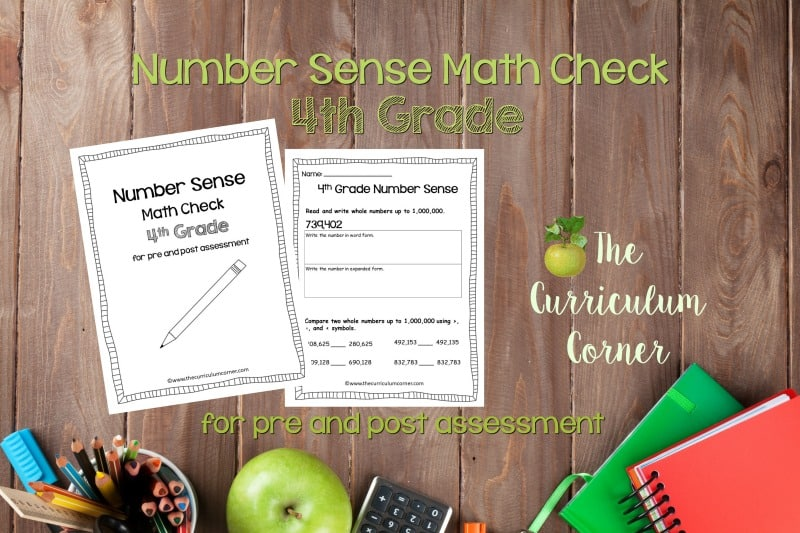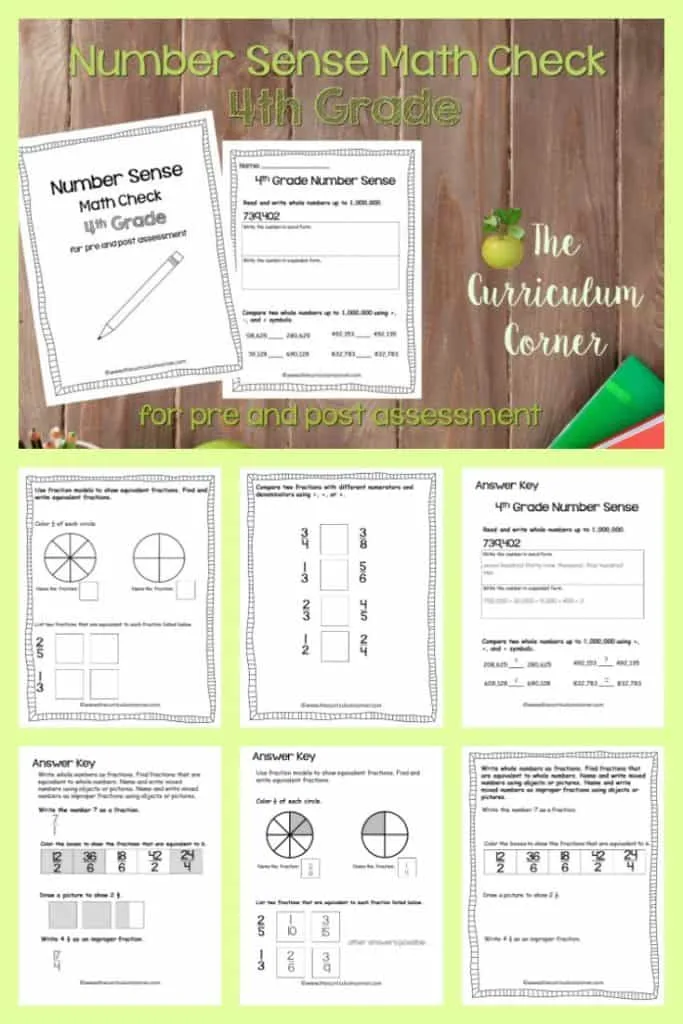# Math Check: 4th Grade Number Sense

## This 4th Grade Number Sense Math Check is designed to be a pre and post assessment for number sense standards in your math classroom.This set of math checks is designed to help you assess fourth grade standards for number sense.

Because states across the nation have adopted a variety of standards, we have created this assessment without indicator numbers. Our hope is that this will make these assessments materials that can be used regardless of the numbers used on your standards.

These assessment pages can be used as pre and post assessment checks to chart growth. You might also use them to check in with your students before a unit test.

Use these problems to help guide your teaching at the beginning of a unit or to help you structure your reteaching groups as you progress.

You will also find an answer key.You will find problems covering each standard along with the standard listed at the top of the page or section.

Problems for the following standards are included:

• Read and write whole numbers up to 1,000,000.
• Compare two whole numbers up to 1,000,000 using >, =, and < symbols.
• Write whole numbers as fractions. Find fractions that are equivalent to whole numbers. Name and write mixed numbers using objects or pictures. Name and write mixed numbers as improper fractions using objects or pictures.
• Use fraction models to show equivalent fractions. Find and write equivalent fractions.
• Compare two fractions with different numerators and denominators using <, =, or >.
• Use words, models, standard form and expanded form to represent decimal numbers to hundredths.
• Find all factor pairs for a whole number between 1 and 100. Determine if a whole number between 1 and 100 is a multiple of a given one-digit number.
• Use place value understanding to round multi-digit whole numbers to any given place value.

The math check created has been designed to touch upon each of the standards.

We will be creating a separate, computation assessment. We do understand that some school districts group beginning number sense with computation. You will be able to pull indicators from each set to fit your school.

We do understand for an end of unit test, you will might need a larger, more in depth test. This resource is not that test. Instead, it is a way for you to take a quick feel of your students understanding of the content.